Скачать презентацию Basic Physical Principles of MRI James Voyvodic Ph

5440a5452022e6f22b9734b4c6b22ab5.ppt

• Количество слайдов: 35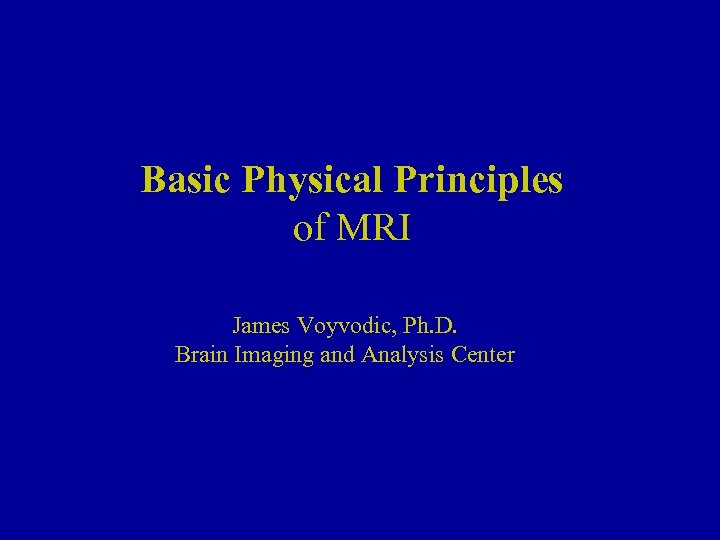Basic Physical Principles of MRI James Voyvodic, Ph. D. Brain Imaging and Analysis Center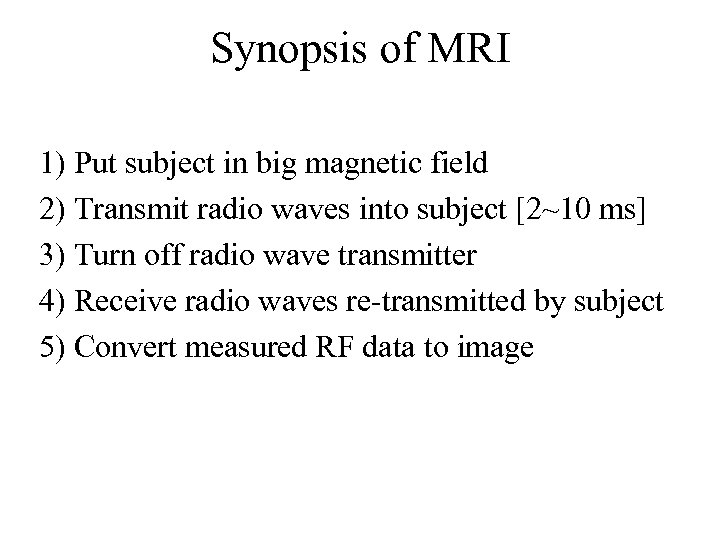Synopsis of MRI 1) Put subject in big magnetic field 2) Transmit radio waves into subject [2~10 ms] 3) Turn off radio wave transmitter 4) Receive radio waves re-transmitted by subject 0 5) Convert measured RF data to image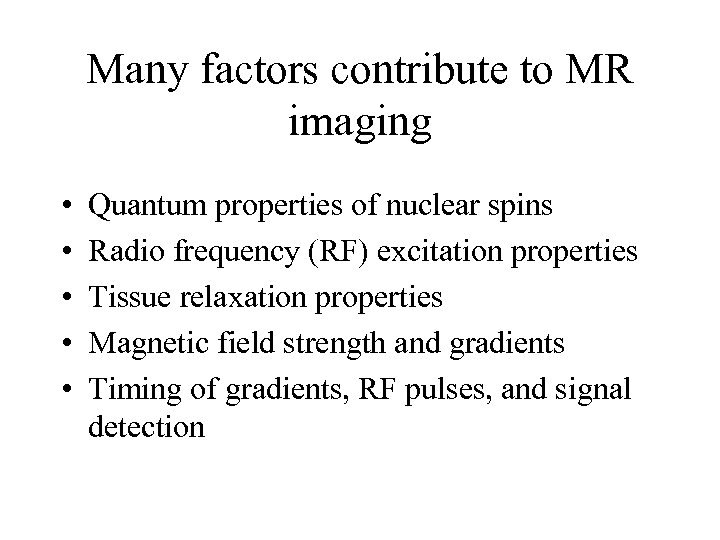Many factors contribute to MR imaging • • • Quantum properties of nuclear spins Radio frequency (RF) excitation properties Tissue relaxation properties Magnetic field strength and gradients Timing of gradients, RF pulses, and signal detection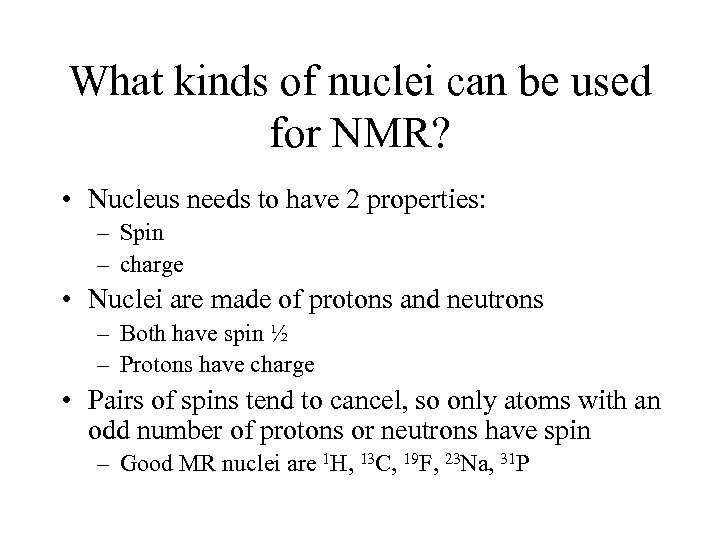What kinds of nuclei can be used for NMR? • Nucleus needs to have 2 properties: – Spin – charge • Nuclei are made of protons and neutrons – Both have spin ½ – Protons have charge • Pairs of spins tend to cancel, so only atoms with an odd number of protons or neutrons have spin – Good MR nuclei are 1 H, 13 C, 19 F, 23 Na, 31 P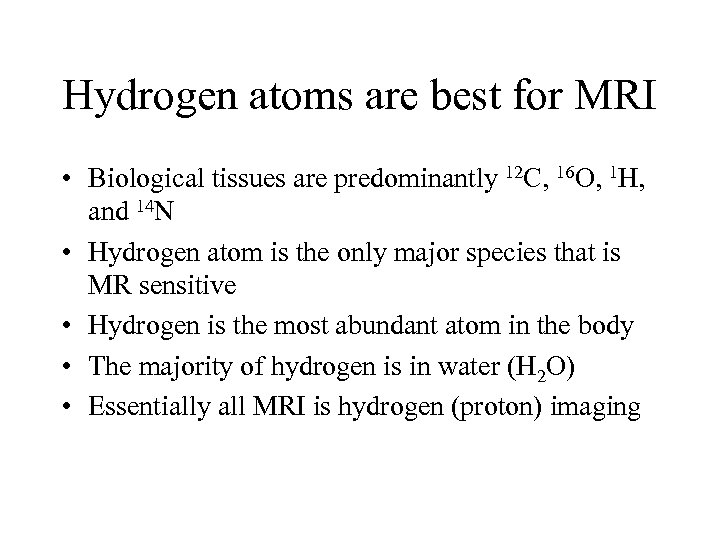Hydrogen atoms are best for MRI • Biological tissues are predominantly 12 C, 16 O, 1 H, and 14 N • Hydrogen atom is the only major species that is MR sensitive • Hydrogen is the most abundant atom in the body • The majority of hydrogen is in water (H 2 O) • Essentially all MRI is hydrogen (proton) imaging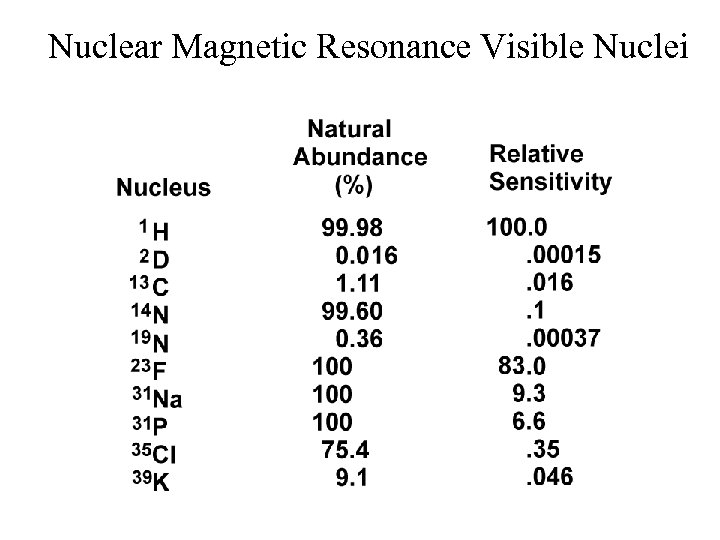Nuclear Magnetic Resonance Visible Nuclei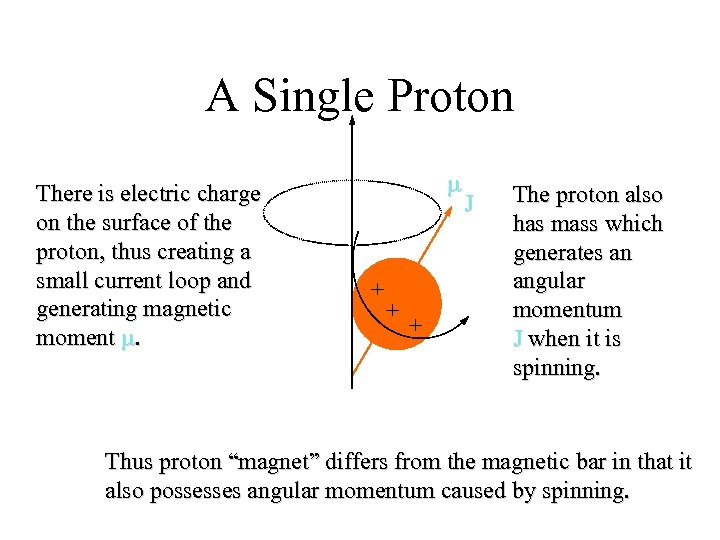A Single Proton There is electric charge on the surface of the proton, thus creating a small current loop and generating magnetic moment m. m + + + J The proton also has mass which generates an angular momentum J when it is spinning. Thus proton “magnet” differs from the magnetic bar in that it also possesses angular momentum caused by spinning.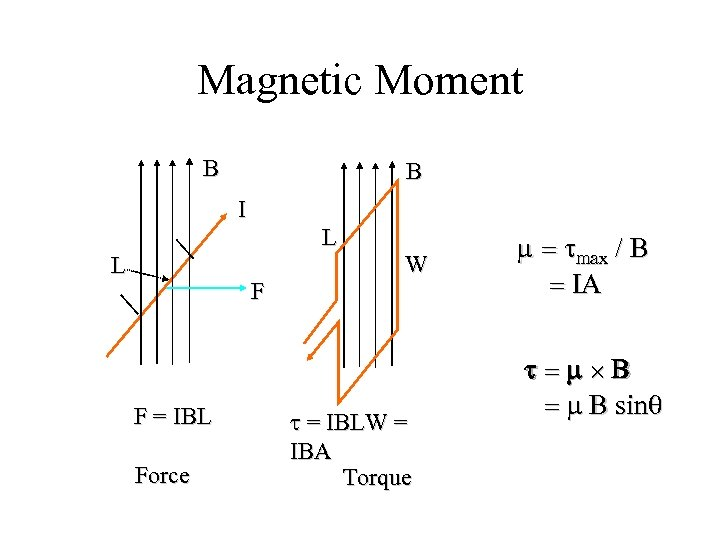Magnetic Moment B B I L L F F = IBL Force W t = IBLW = IBA Torque m = tmax / B = IA t=m B = m B sinq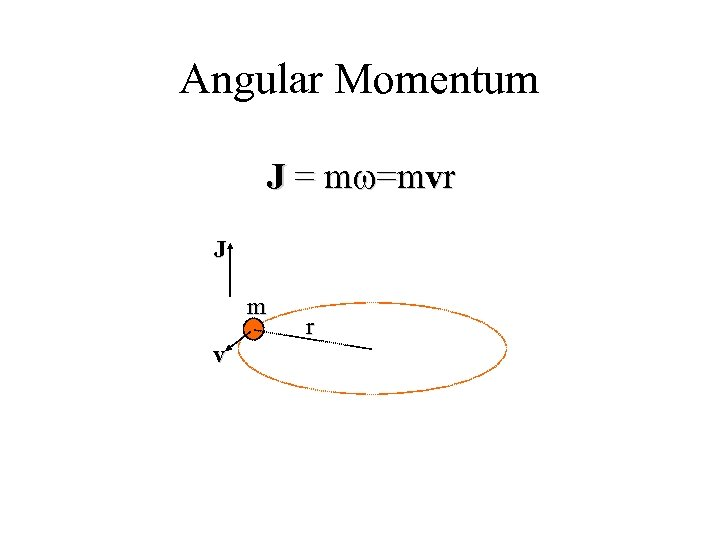Angular Momentum J = mw=mvr J m v r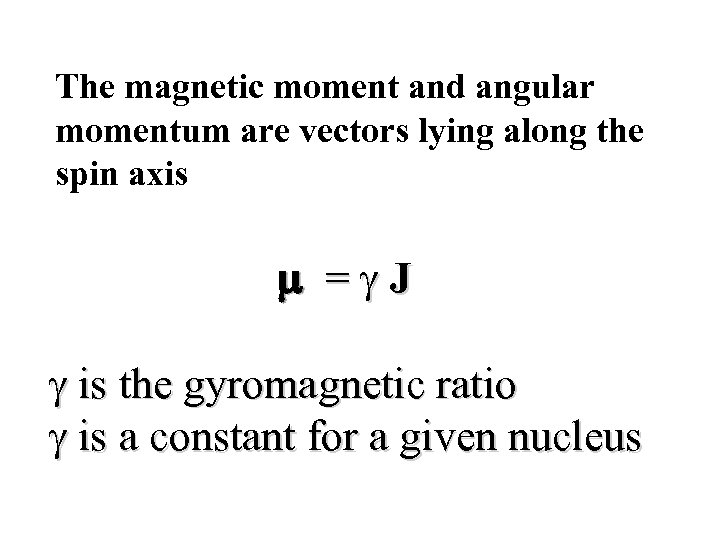The magnetic moment and angular momentum are vectors lying along the spin axis m =g. J g is the gyromagnetic ratio g is a constant for a given nucleus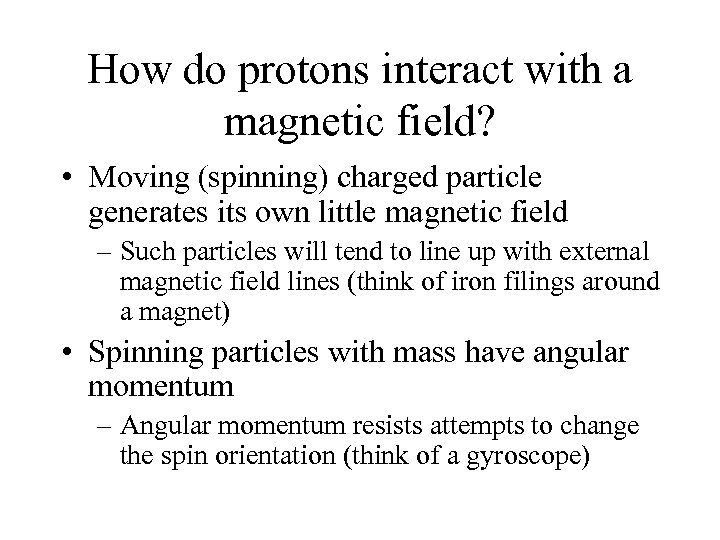How do protons interact with a magnetic field? • Moving (spinning) charged particle generates its own little magnetic field – Such particles will tend to line up with external magnetic field lines (think of iron filings around a magnet) • Spinning particles with mass have angular momentum – Angular momentum resists attempts to change the spin orientation (think of a gyroscope)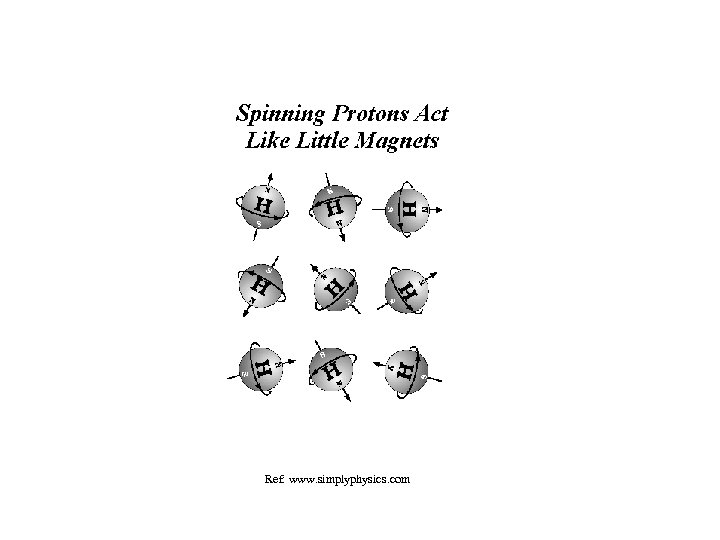Ref: www. simplyphysics. com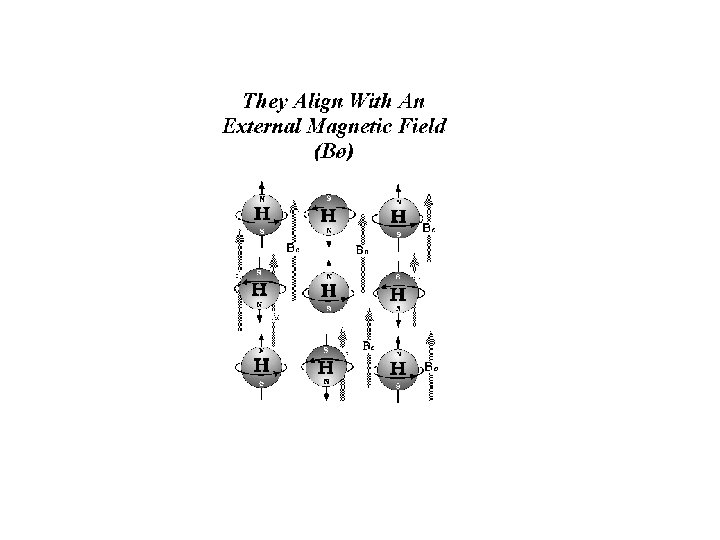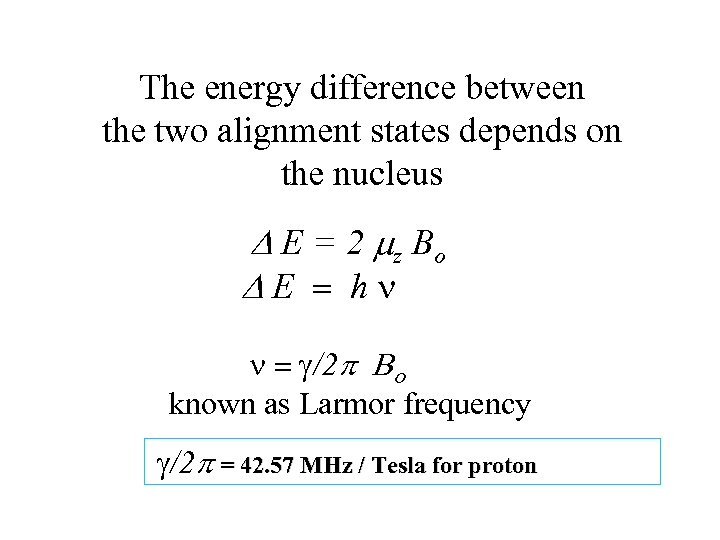The energy difference between the two alignment states depends on the nucleus D E = 2 mz B o DE = hn n = g/2 p Bo known as Larmor frequency g/2 p = 42. 57 MHz / Tesla for proton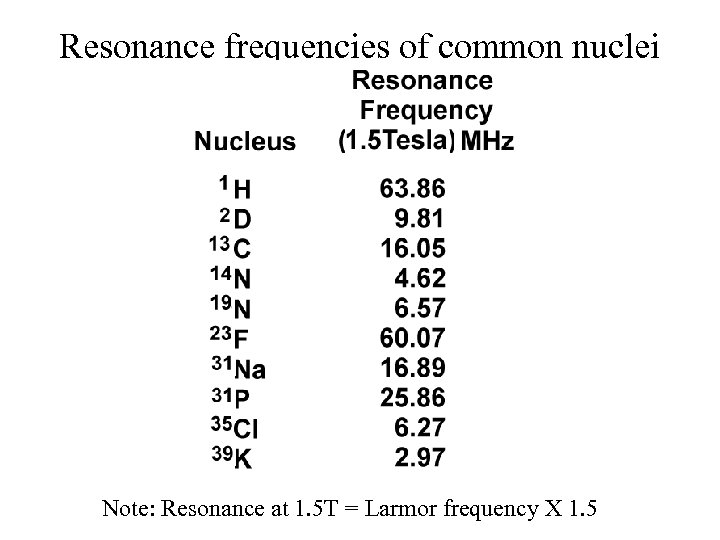Resonance frequencies of common nuclei Note: Resonance at 1. 5 T = Larmor frequency X 1. 5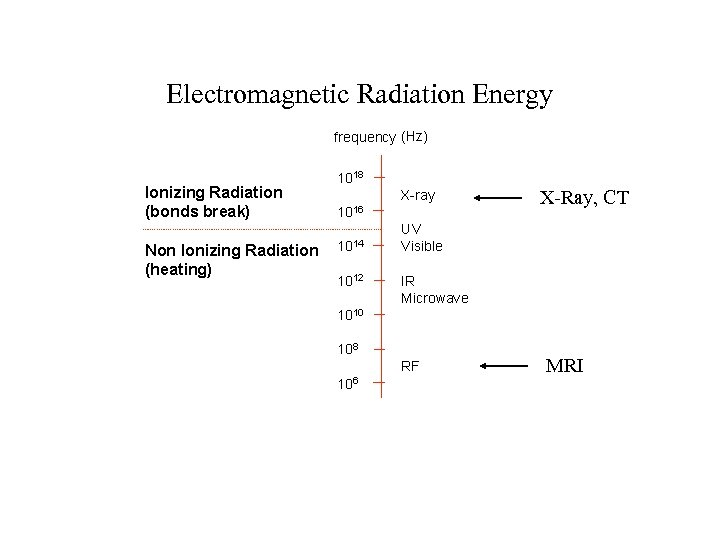Electromagnetic Radiation Energy X-Ray, CT MRI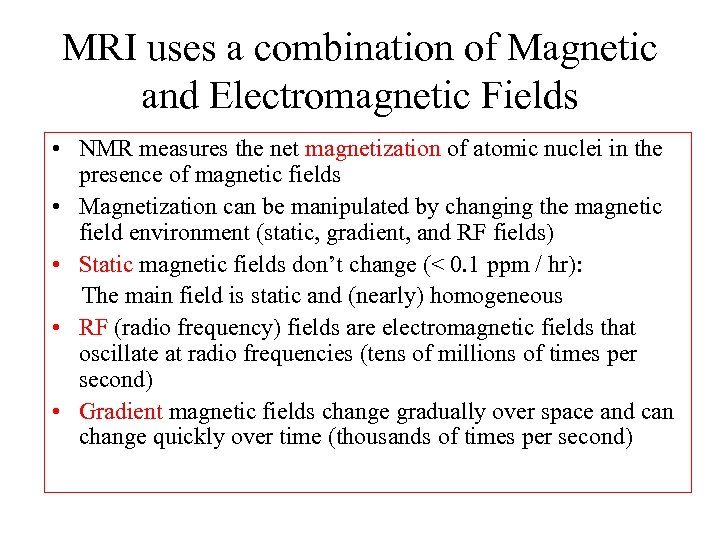MRI uses a combination of Magnetic and Electromagnetic Fields • NMR measures the net magnetization of atomic nuclei in the presence of magnetic fields • Magnetization can be manipulated by changing the magnetic field environment (static, gradient, and RF fields) • Static magnetic fields don’t change (< 0. 1 ppm / hr): The main field is static and (nearly) homogeneous • RF (radio frequency) fields are electromagnetic fields that oscillate at radio frequencies (tens of millions of times per second) • Gradient magnetic fields change gradually over space and can change quickly over time (thousands of times per second)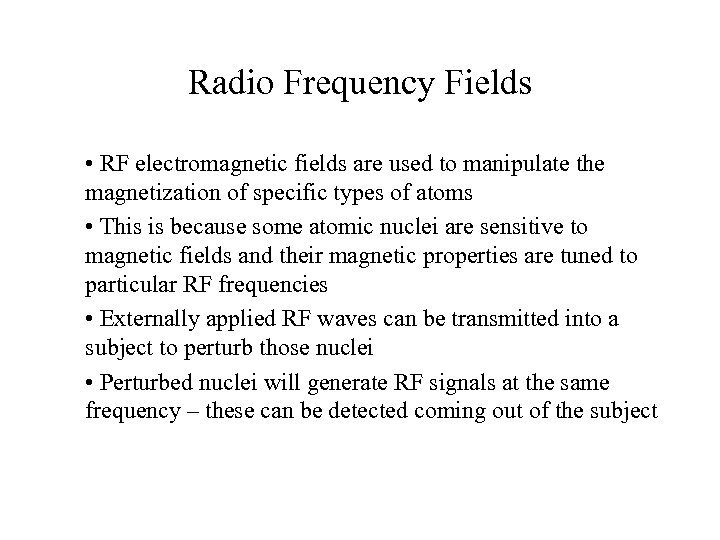Radio Frequency Fields • RF electromagnetic fields are used to manipulate the magnetization of specific types of atoms • This is because some atomic nuclei are sensitive to magnetic fields and their magnetic properties are tuned to particular RF frequencies • Externally applied RF waves can be transmitted into a subject to perturb those nuclei • Perturbed nuclei will generate RF signals at the same frequency – these can be detected coming out of the subject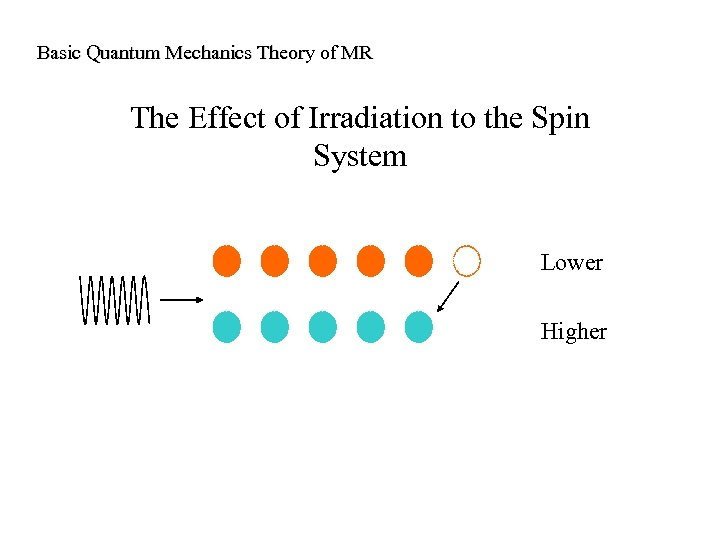Basic Quantum Mechanics Theory of MR The Effect of Irradiation to the Spin System Lower Higher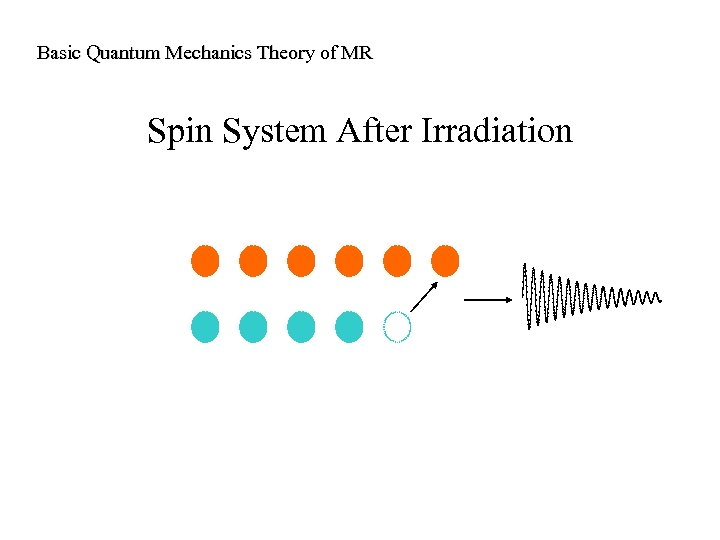Basic Quantum Mechanics Theory of MR Spin System After Irradiation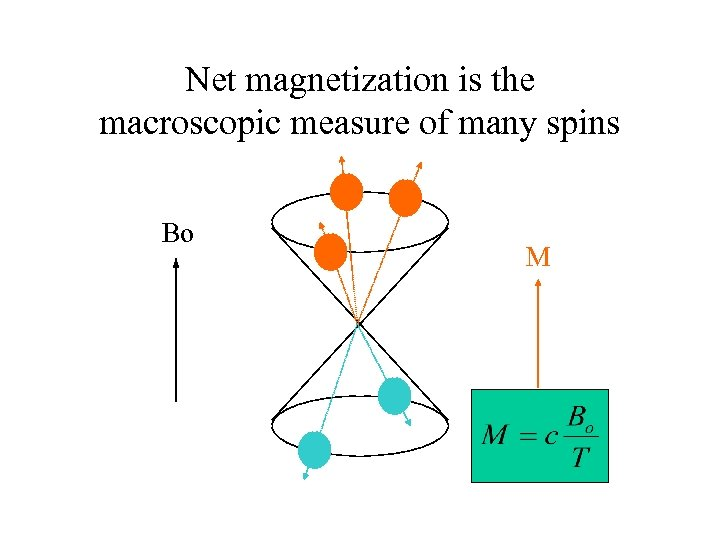Net magnetization is the macroscopic measure of many spins Bo M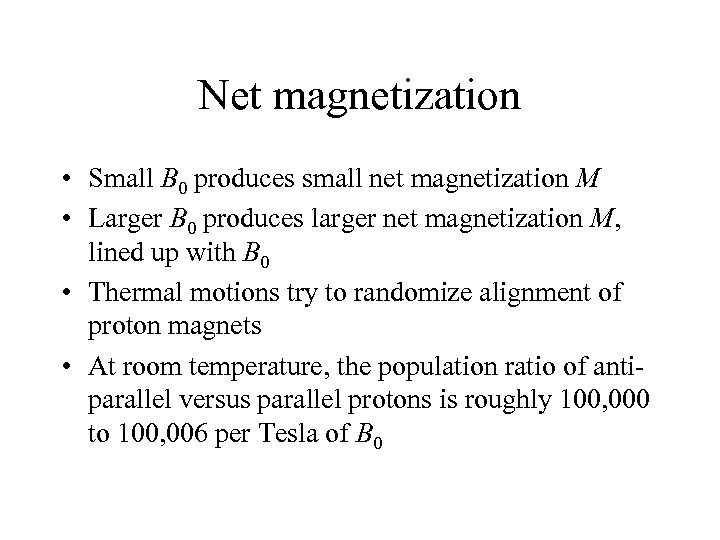Net magnetization • Small B 0 produces small net magnetization M • Larger B 0 produces larger net magnetization M, lined up with B 0 • Thermal motions try to randomize alignment of proton magnets • At room temperature, the population ratio of antiparallel versus parallel protons is roughly 100, 000 to 100, 006 per Tesla of B 0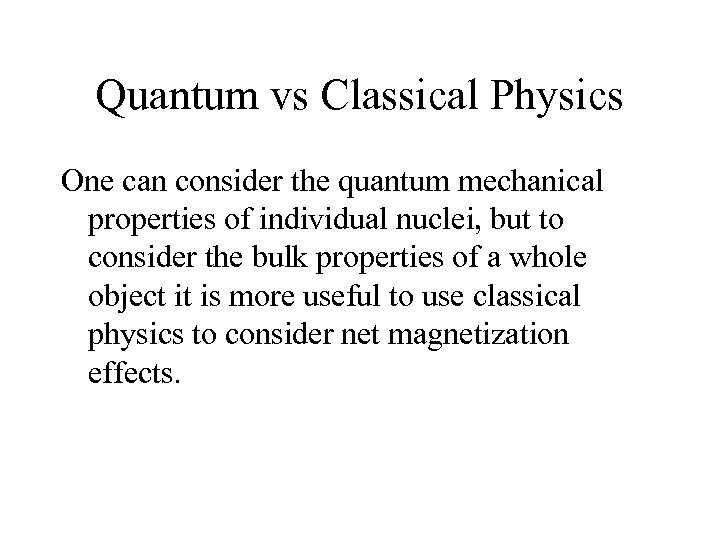Quantum vs Classical Physics One can consider the quantum mechanical properties of individual nuclei, but to consider the bulk properties of a whole object it is more useful to use classical physics to consider net magnetization effects.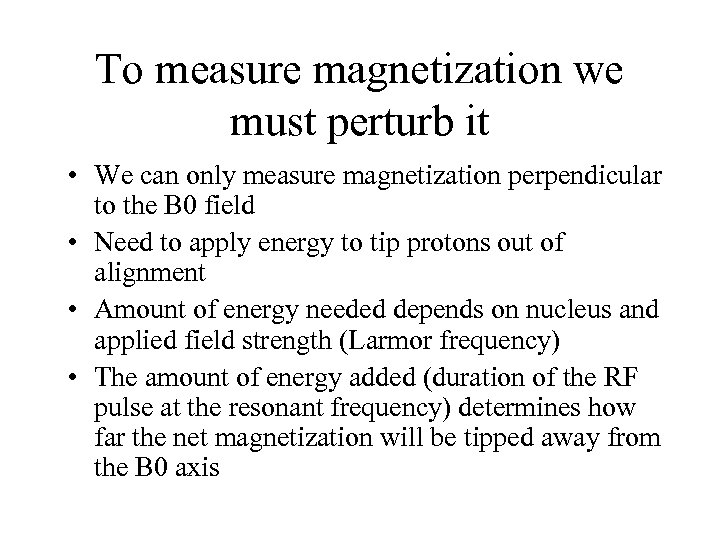To measure magnetization we must perturb it • We can only measure magnetization perpendicular to the B 0 field • Need to apply energy to tip protons out of alignment • Amount of energy needed depends on nucleus and applied field strength (Larmor frequency) • The amount of energy added (duration of the RF pulse at the resonant frequency) determines how far the net magnetization will be tipped away from the B 0 axis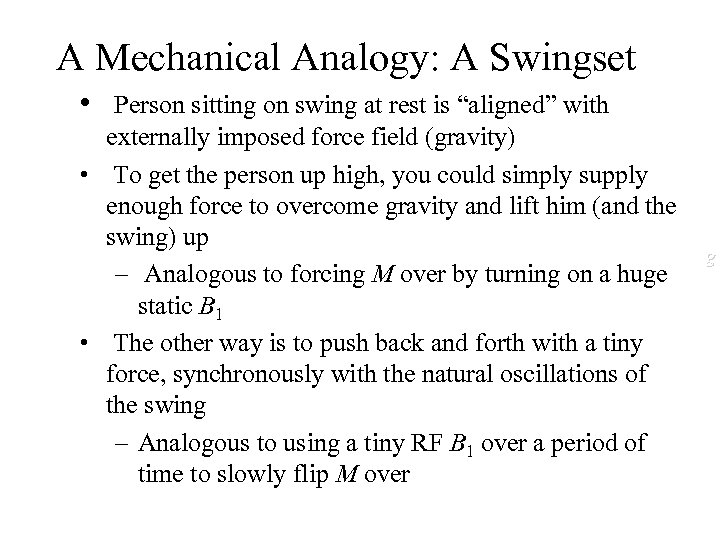A Mechanical Analogy: A Swingset • Person sitting on swing at rest is “aligned” with externally imposed force field (gravity) • To get the person up high, you could simply supply enough force to overcome gravity and lift him (and the swing) up – Analogous to forcing M over by turning on a huge static B 1 • The other way is to push back and forth with a tiny force, synchronously with the natural oscillations of the swing – Analogous to using a tiny RF B 1 over a period of time to slowly flip M over g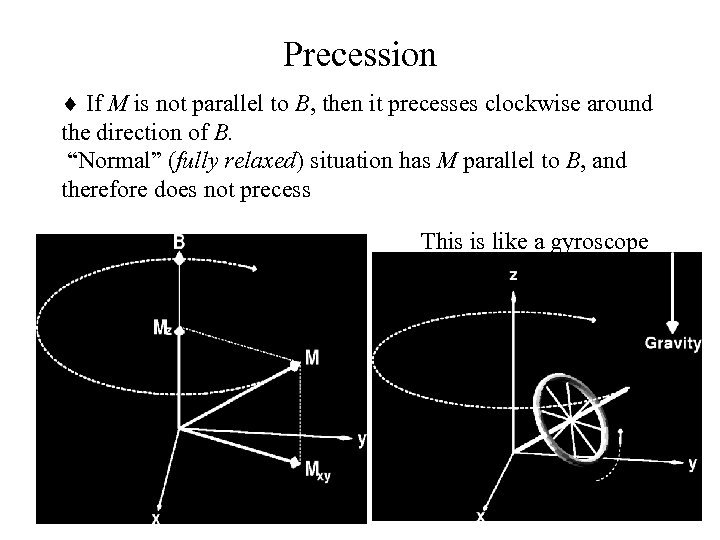Precession ¨ If M is not parallel to B, then it precesses clockwise around the direction of B. “Normal” (fully relaxed) situation has M parallel to B, and therefore does not precess This is like a gyroscope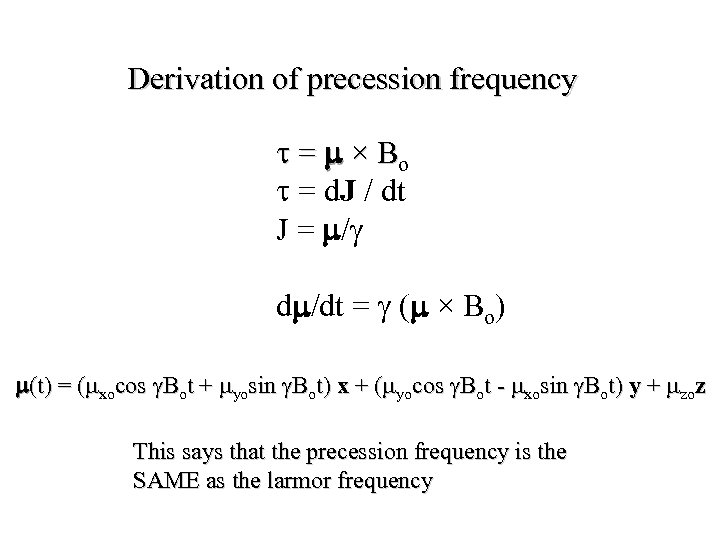Derivation of precession frequency t = m × Bo t = d. J / dt J = m/g dm/dt = g (m × Bo) m(t) = (mxocos g. Bot + myosin g. Bot) x + (myocos g. Bot - mxosin g. Bot) y + mzoz This says that the precession frequency is the SAME as the larmor frequency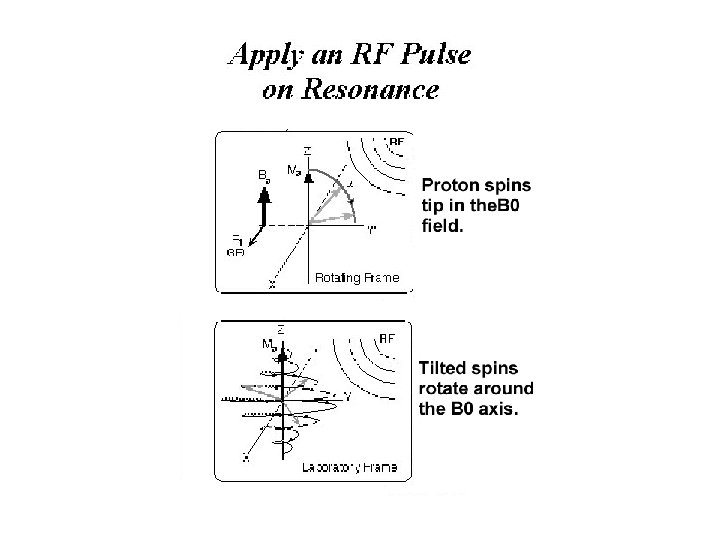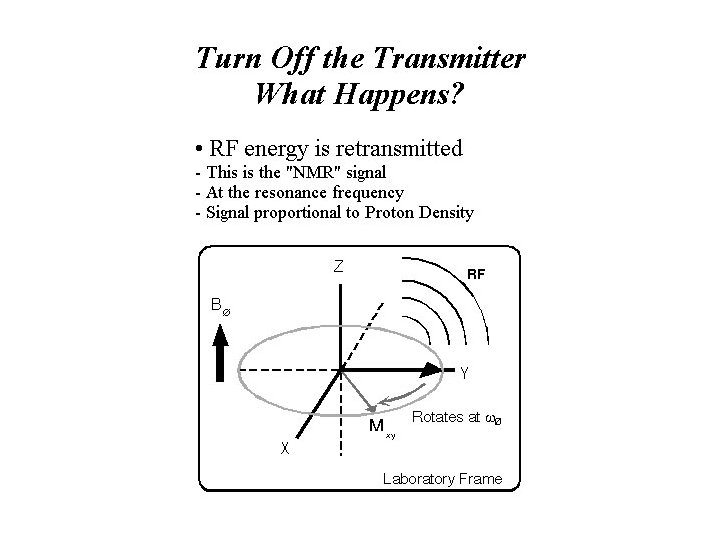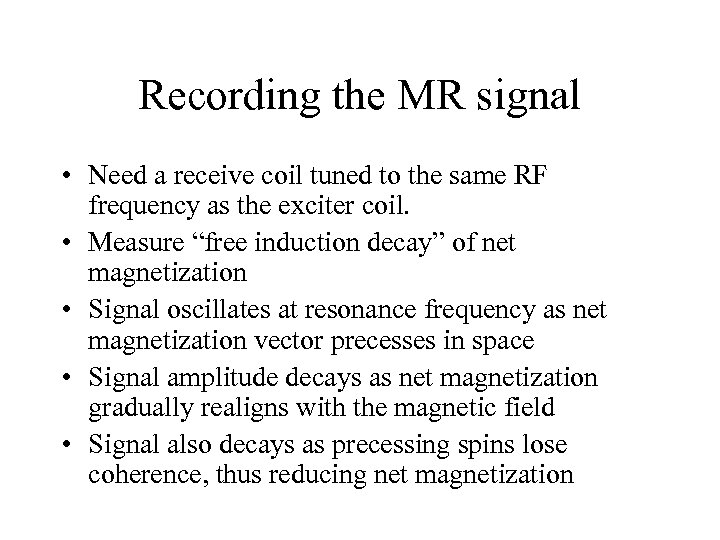Recording the MR signal • Need a receive coil tuned to the same RF frequency as the exciter coil. • Measure “free induction decay” of net magnetization • Signal oscillates at resonance frequency as net magnetization vector precesses in space • Signal amplitude decays as net magnetization gradually realigns with the magnetic field • Signal also decays as precessing spins lose coherence, thus reducing net magnetization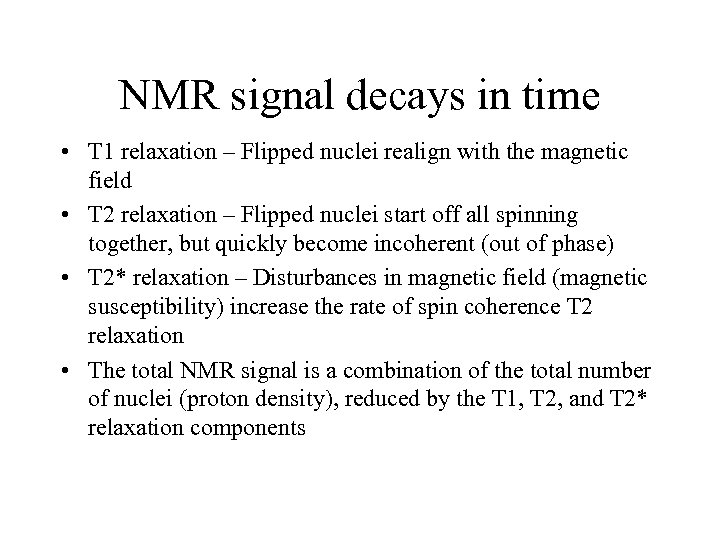NMR signal decays in time • T 1 relaxation – Flipped nuclei realign with the magnetic field • T 2 relaxation – Flipped nuclei start off all spinning together, but quickly become incoherent (out of phase) • T 2* relaxation – Disturbances in magnetic field (magnetic susceptibility) increase the rate of spin coherence T 2 relaxation • The total NMR signal is a combination of the total number of nuclei (proton density), reduced by the T 1, T 2, and T 2* relaxation components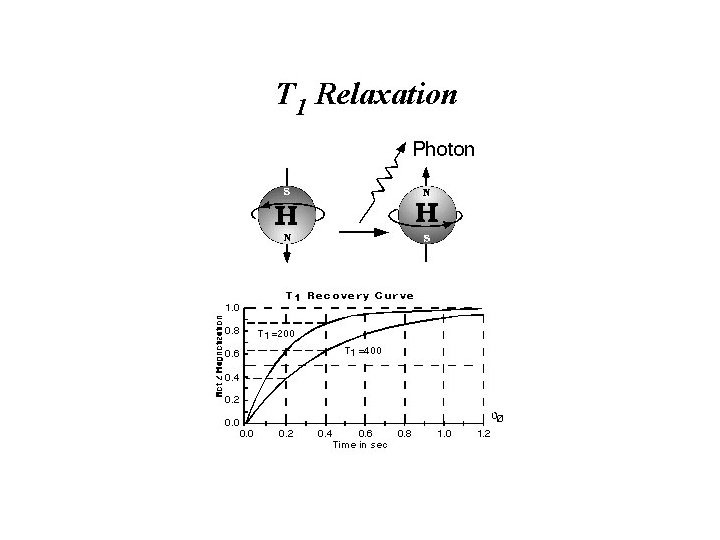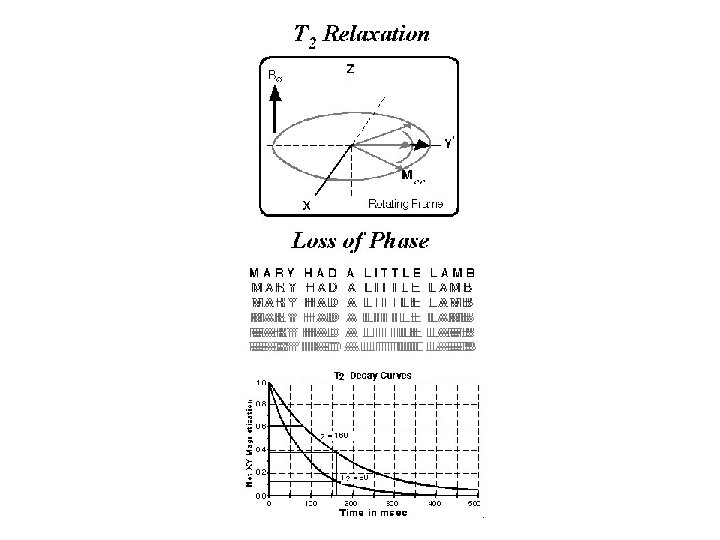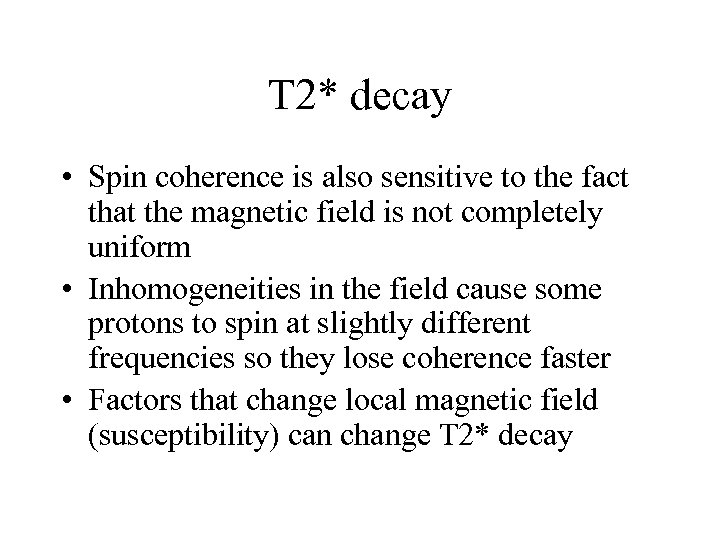T 2* decay • Spin coherence is also sensitive to the fact that the magnetic field is not completely uniform • Inhomogeneities in the field cause some protons to spin at slightly different frequencies so they lose coherence faster • Factors that change local magnetic field (susceptibility) can change T 2* decay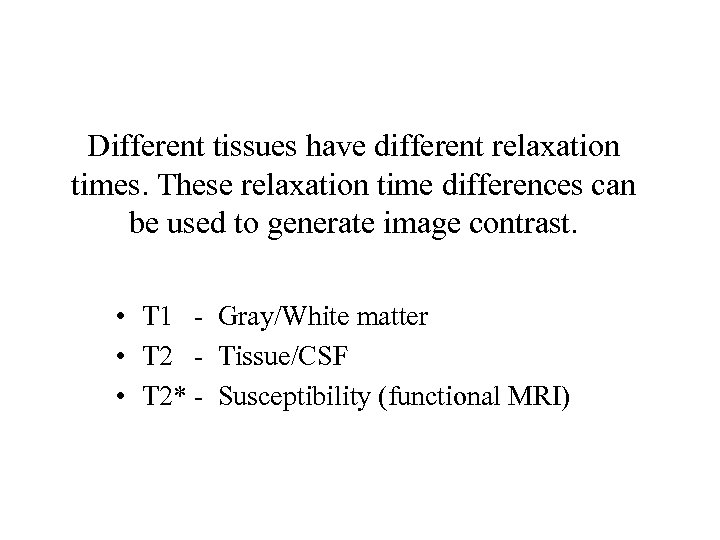Different tissues have different relaxation times. These relaxation time differences can be used to generate image contrast. • T 1 - Gray/White matter • T 2 - Tissue/CSF • T 2* - Susceptibility (functional MRI)